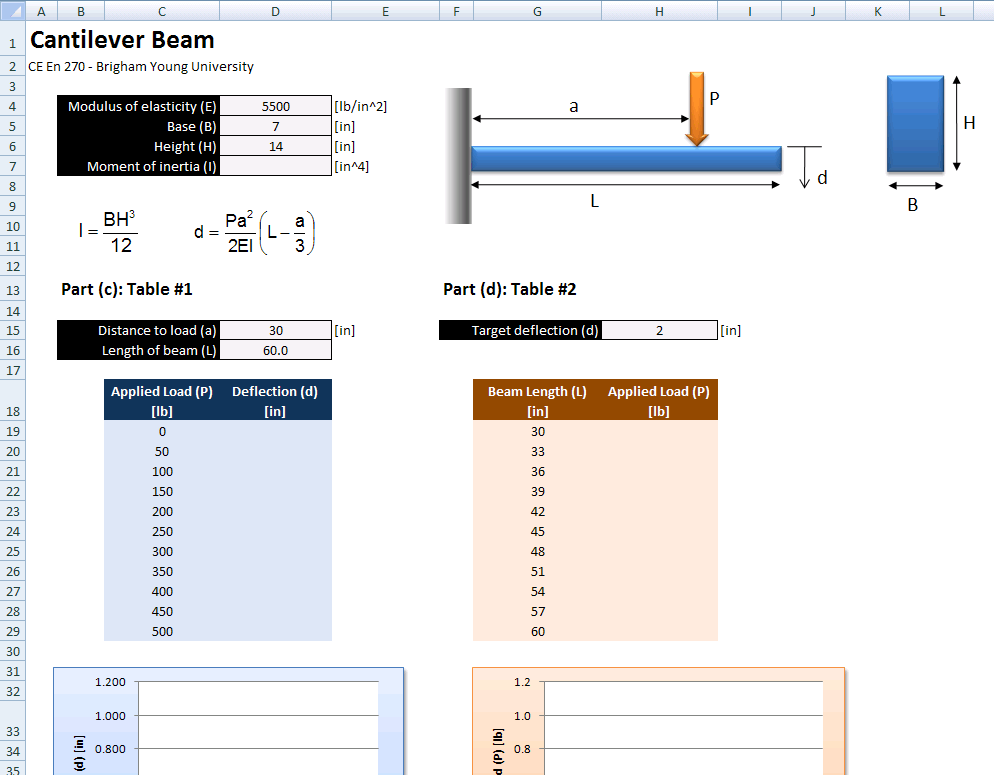# HW#8 - Cantilever Beam

In structural engineering applications, it is often necessary to predict the deflection at the tip of a cantilever beam. Write a spreadsheet to compute the deflection of a cantilever beam using the following parameters and equations: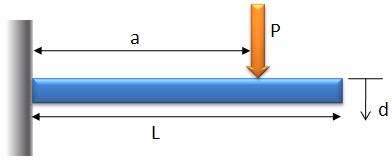Beam cross-section: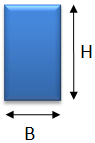where:

 P = applied load a = length from fixed end to applied load E = modulus of elasticity of beam material I = moment of inertia of the beam =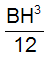d = deflection of beam =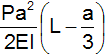Known quantities:

 E = 5500 lb/in2 B = 7 in H = 14 in

Problem:

Write a spreadsheet to compute the deflection of the tip of the beam as a function of the applied load. The spreadsheet should have the following features:

a. Create a drawing of the cantilever beam as shown above using the built-in drawing tools in Excel. Do not copy and embed the drawing shown above.

b. Use the Equation Editor (Insert|Symbols|Equation) to add a drawing of the equations shown above for the moment of inertia and beam deflection.

c. Assume that a = 30 in and length = 60 in. Make a table showing deflection vs. P. Let P vary from 0 - 500 lbs in increments of 50 lbs. Make a plot showing deflection vs. P.

d. Solve the deflection equation shown above for P. Add another table to your spreadsheet to compute the load (P) which would result in a deflection of 2 in assuming that the load is applied at the end of the beam (a = L) and the beam length varies from 30 in to 60 in by increments of 3 in. Make a graph of your solution (P vs. L). Note: Do not use a from the previous table!

In summary, you may want to lay out your spreadsheet something like this: